Straight to the Tree： Constituency Parsing with Neural Syntactic Distance# 摘要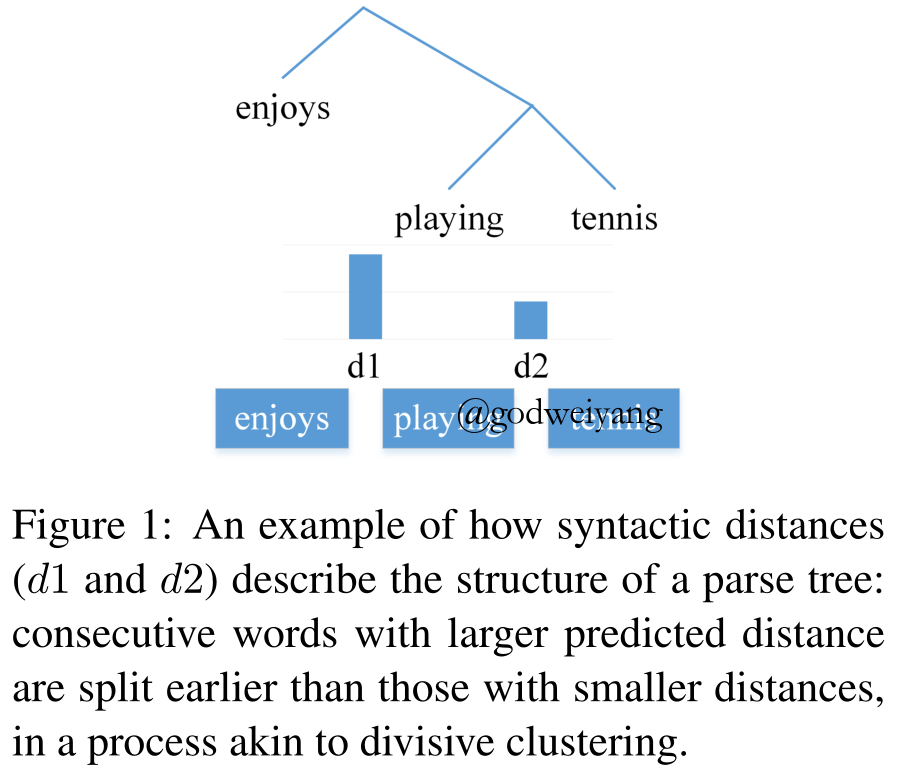# Syntactic Distances

$sign({d_i} - {d_j}) = sign(\tilde d_i^{i - 1} - \tilde d_j^{j - 1})$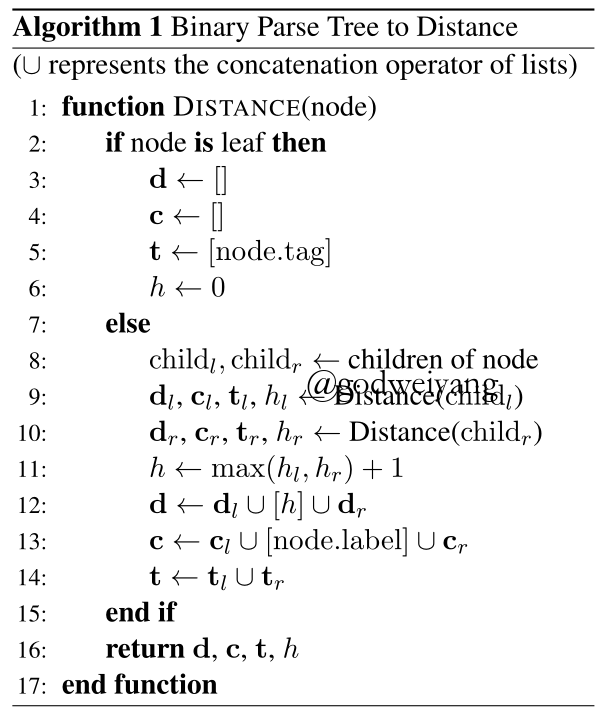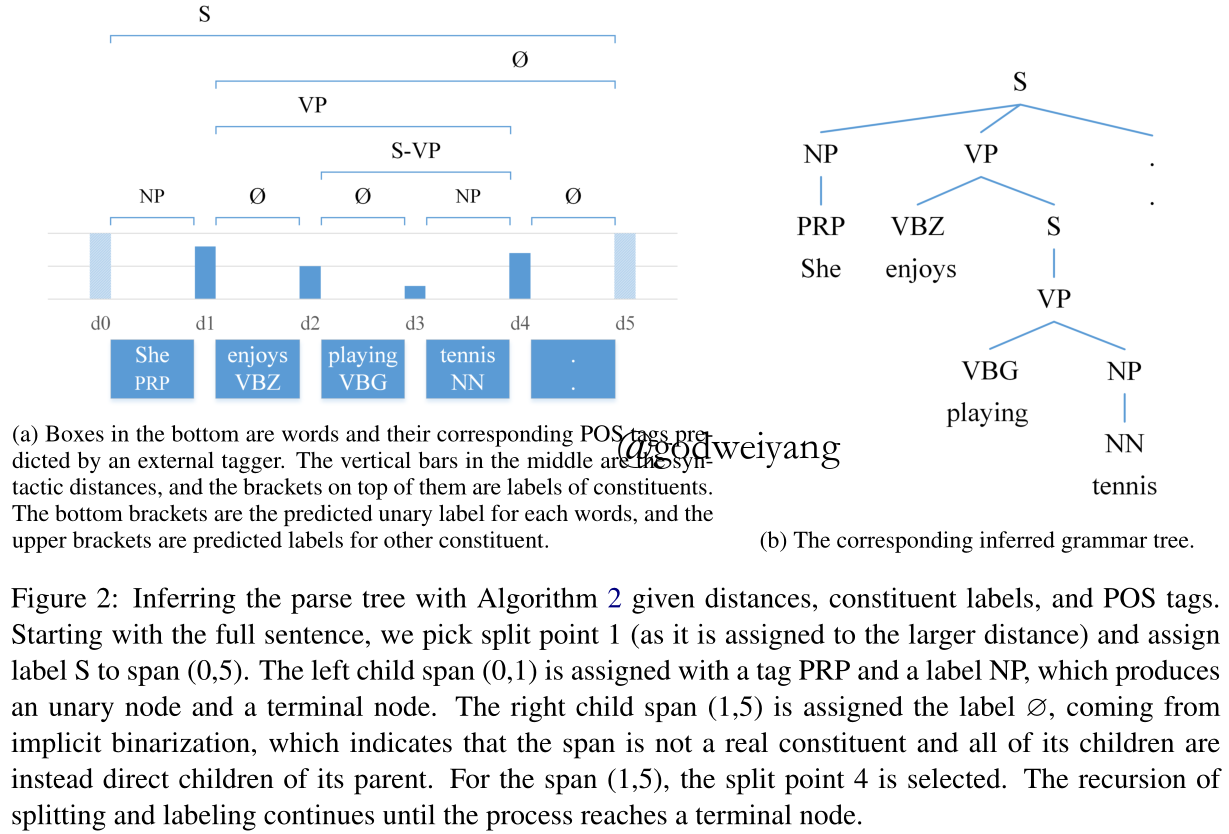# 训练

## 模型结构

$h_0^w, \ldots ,h_n^w = {\rm{BiLST}}{ {\rm{M}}_w}([e_0^w,e_0^t], \ldots ,[e_n^w,e_n^t])$

$p(c_i^w|w) = {\rm{softmax(FF}}_c^w(h_i^w))$

$g_1^s, \ldots ,g_n^s = {\rm{CONV(}}h_0^w, \ldots ,h_n^w)$

$h_1^s, \ldots ,h_n^s = {\rm{BiLST}}{ {\rm{M}}_s}(g_1^s, \ldots ,g_n^s)$

${ {\hat d}_i} = {\rm{F}}{ {\rm{F}}_d}(h_i^s)$

$p(c_i^s|w) = {\rm{softmax(FF}}_c^s(h_i^s))$

## 损失函数

$L_{dist}^{mse} = \sum\limits_i { { {({d_i} - { {\hat d}_i})}^2}}$

$L_{dist}^{rank} = \sum\limits_{i,j > i} {\max (0,1 - sign({d_i} - {d_j})({ {\hat d}_i} - { {\hat d}_j}))}$

$L = {L_{ {\rm{label}}}} + L_{dist}^{rank}$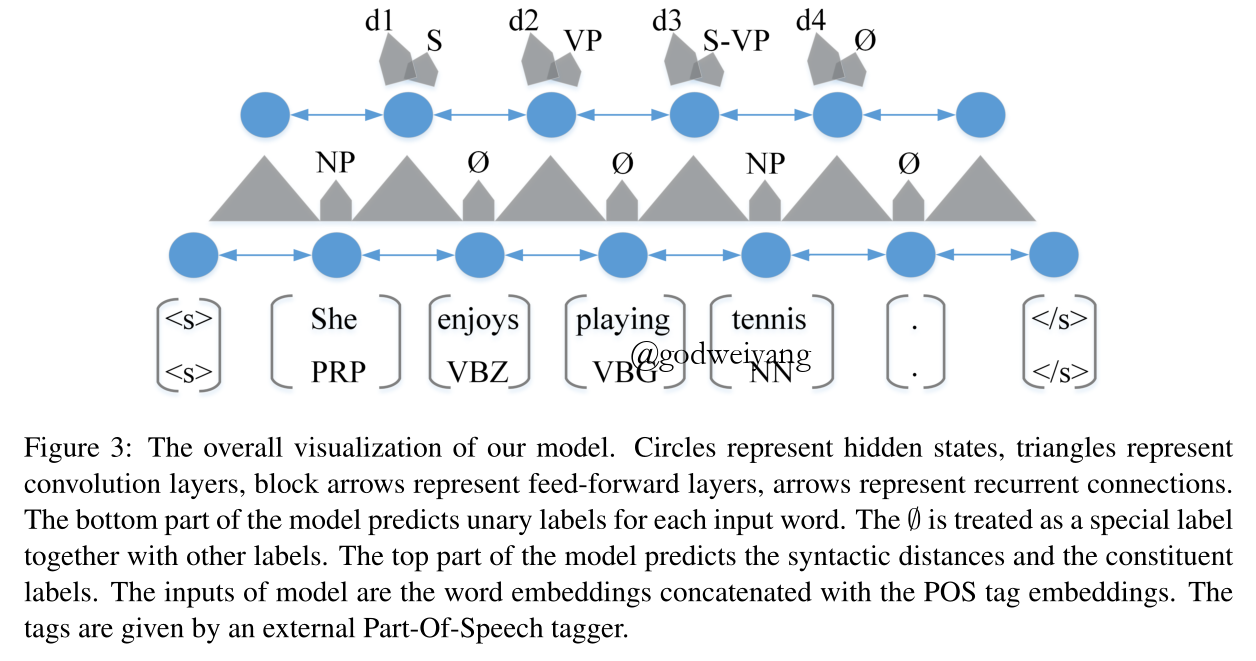# 实验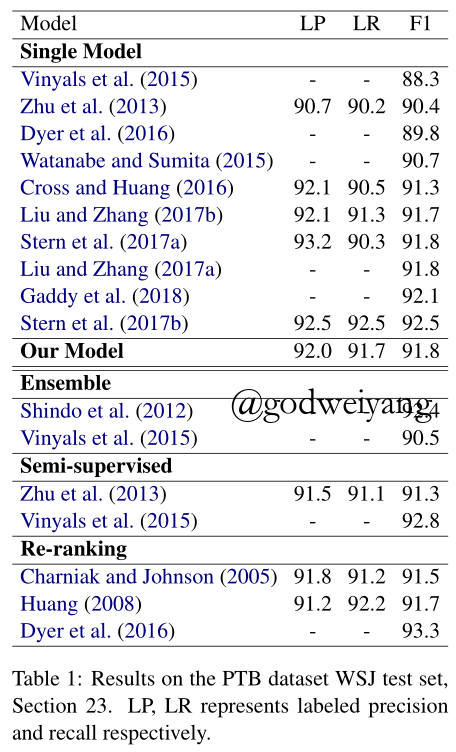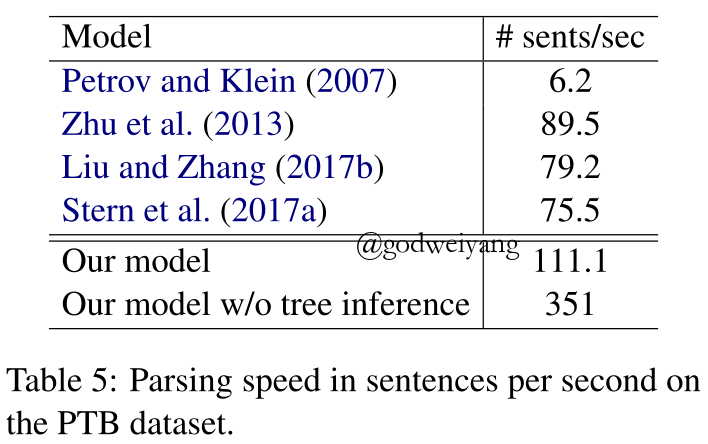# 总结

• 可以将LSTM替换为self-attention，因为之前博客讲到了，伯克利的self-attention编码器比LSTM编码器准确率高了1个多的百分点。
• 可以尝试最近新出的词向量模型ELMo，也许会有特别大的提升。
• 加入词级别的Char-LSTM，可能会有一定提升。
• 我觉得split的表示可以更加复杂化一点，而不仅仅是一层卷积+一层LSTM这么简单。

转载规则

《Straight to the Tree： Constituency Parsing with Neural Syntactic Distance》 韦阳 采用 知识共享署名 4.0 国际许可协议 进行许可。
上一篇Deep Understanding of Dynamic Oracle in Constituent Parsing

2018-08-03Constituency Parsing with a Self-Attentive Encoder

2018-07-04
目录